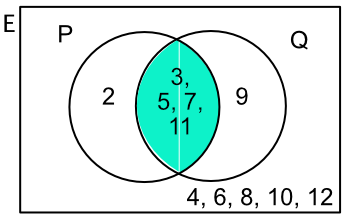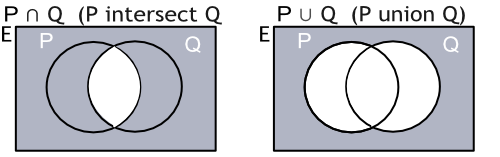Venn Diagrams

## Venn Diagrams

Venn Diagrams are used to display sets. A Venn diagram consists of one or more circles within a rectangle.

The rectangle defines the Universal Set, and each circle within the rectangle is a different set. Elements not in any set are shown within the rectangle but not inside a circle.

For example, if two dice are thrown then ε = {2,3,4,5,6,7,8,9,10,11,12). Set P consists of Prime Numbers and set Q consists of odd numbers:Venn Diagrams can be used to determine the interaction of sets. The areas of white in the diagrams below show the result of the operation:P nn Q = {3, 5, 7, 11} and P uu Q = {2, 3, 5, 7, 9, 11}.

Venn diagrams can also be used to show totals, rather than individual items.

## Example 1

Given a set of integers from 1 to 15, set A = {multiples of two} and set B = {multiples of three}. The Venn Diagram has been started. Show the elements of A ∩ B.A={2,4,6,8,10,12,14} and B={3,6,9,12,15}

Part x is A overlapping B, which is A nn B = {6, 12}.

Answer: A nn B = {6, 12}

## Example 2

A field trip consists of 30 students. 8 of these students do not study a language.

21 students study French. 18 students study German. Complete the Venn Diagram, finding values for w, x, y and z.The 8 students that do not form part of any set are shown outside the sets in position w.

The total for French and German is 21 + 18 = 39, but there are only 22 students that study a language. The number of students studying two languages is 39 - 22 = 17 = y.

There are 21 students studying French less the 17 studying both = 4 = x.

Similarly for German: 18 - 17 = 1 = z.

Check it: w + x + y + z = 30✔

Answer: w = 8, x = 4, y = 17, z = 1